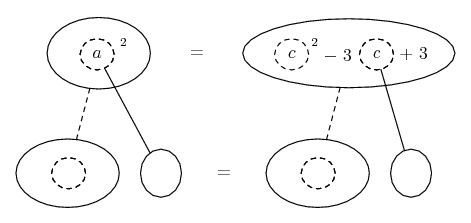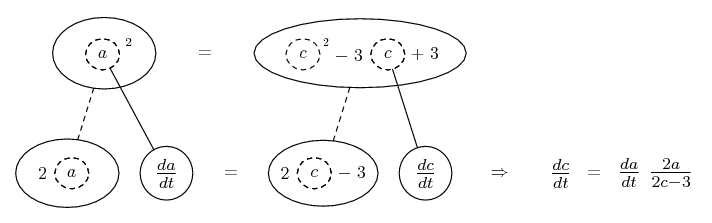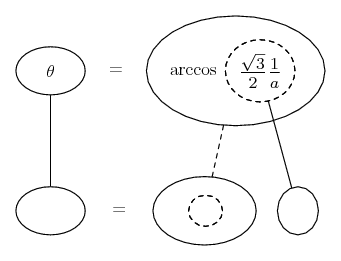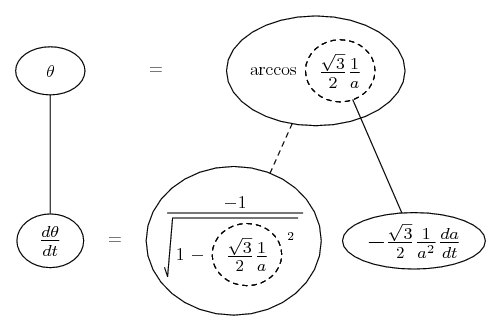# Thread: trignometric differentiation

1. ##trignometric differentiation

An aircraft climbing at a constant angle of 30
above the horizontal passes directly over a ground radar station at an altitude of 1 km. At a later instant, the radar shows that the aircraft is at an air distance of 2 km from the station, and that this distance is increasing at 7 km/min. What is the speed of the aircraft at that instant? [Note: the law of cosines may be useful.] At what rate is the angle between the vertical line directly above the station and the line from the station to the aircraft changing?

--->I found the angle to be 34. To find the velocity at that point do I need to first find the distance and then differentiate the cosine law? Also, please guide me through the rate of change of angle.

2. Related rates nearly always depend on the chain rule...... where straight continuous lines differentiate downwards (integrate up) with respect to the main variable (in this case time), and the straight dashed line similarly but with respect to the dashed balloon expression (the inner function of the composite which is subject to the chain rule).

Call the angle of take-off A. Call the ground (between take-off point and station) b, and call the distance flown c. Also, call the side which is opposite (opposite the angle A) a.

tan(30) being one over root 3, we have b = root 3.

Then the cosine rule

$\displaystyle \cos A = \frac{b^2 + c^2 - a^2}{2bc}$

gives us

$\displaystyle \frac{\sqrt{3}}{2} = \frac{(\sqrt{3})^2 + c^2 - a^2}{2 * \sqrt{3} * c}$

So we have a relationship between a and c...Differentiate with respect to the inner function, and the inner function with respect to t. Then sub in a = 2, da/dt = 7, and also c when you've found it from solving

$\displaystyle 2^2 = c^2 - 3c + 3$

(by completing the square).

Spoiler:Next we want to get the angle made by side a as a function of the length of side a. They said the angle relative to the vertical, but all they want is the rate of change, and this will be the same as the rate of change of the angle between a and, say, the perpendicular from the station to the flight path (this terminology possibly lacking - will amend). This angle - call it theta - is an easier one to get from length a, unless I'm wrong on this point.

Anyway, the perpendicular has length root 3 over 2 (work this out on your diagram). So...

$\displaystyle \cos \theta = \frac{\sqrt{3}}{2}\frac{1}{a}$

So...Again, you just need to differentiate with respect to time, and sub in the values for a and da/dt.

Spoiler:Hope this helps.

__________________________________________

Don't integrate - balloontegrate!

Balloon Calculus: Gallery

Balloon Calculus Drawing with LaTeX and Asymptote!

#### Search Tags

differentiation, trignometric